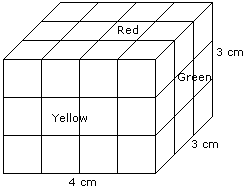# Verbal Reasoning - Cube and Cuboid - Discussion

The following questions are based on the information given below:

1. There is a cuboid whose dimensions are 4 x 3 x 3 cm.
2. The opposite faces of dimensions 4 x 3 are coloured yellow.
3. The opposite faces of other dimensions 4 x 3 are coloured red.
4. The opposite faces of dimensions 3 x 3 are coloured green.
5. Now the cuboid is cut into small cubes of side 1 cm.

4.

How many small cubes will have only one face coloured ?

 [A]. 10 [B]. 12 [C]. 14 [D]. 18

Explanation:Number of small cubes having only one face coloured = 2 x 2 + 2 x 2 + 2 x 1

= 4 + 4 + 2

= 10

 Roshan said: (Nov 7, 2011) Can someone explain it more clearly ?

 Dhana said: (Jan 9, 2012) I did not understand.

 Gaurav said: (Sep 28, 2013) For red 2 from top and 2 from bottom. For green 1 from side and 1 from backside. For yellow 2 from front and 2 from back. Therefore, 4+2+4=10.

 Rahim said: (Dec 2, 2014) Can we use any formula?

 Sabiar said: (May 12, 2016) Anybody give me the actual formula to solve this problem.

 Mahesh said: (Nov 21, 2016) The new l,b,h is (L-2),(b-2),(h-2), then perform lb +bh+hl. After that multiply by 2 for this question new l,b,h after subtracting it from 2 is, 2, 1, 2....2 * 1 + 1 * 1 + 1* 2 = 5 multiply it by two (5 * 2 = 10 ans).

 Alina said: (Dec 13, 2017) We can simplify it as; 5 [(l-2). (b-2) +(b-2). (h-2)+(h-2). (l-2)] . *this formula is applied only if the small cube formed has its side as unity.